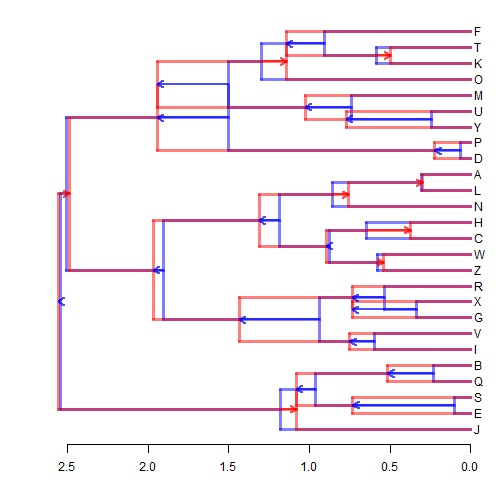## Thursday, May 4, 2017

### Plotting alternative time trees with arrows between corresponding nodes

Today
Mike Alfaro challenged me to generate a plot with two time trees of matching topology using arrows to show the timing difference for divergence events between the two alternative reconstructions.

Here's how to do it very simply using phytools in R:

``````library(phytools)
h1<-max(nodeHeights(t1))
h2<-max(nodeHeights(t2))
plotTree(if(h1>h2) t1 else t2,plot=FALSE,mar=c(4.1,1.1,1.1,1.1))
``````
``````xlim<-get("last_plot.phylo",envir=.PlotPhyloEnv)\$x.lim[2:1]
plotTree(t1,color=make.transparent("blue",0.5),mar=c(4.1,1.1,1.1,1.1),
xlim=xlim,direction="leftwards",lwd=3)
T1<-get("last_plot.phylo",envir=.PlotPhyloEnv)
axis(1)
plotTree(t2,color=make.transparent("red",0.5),mar=c(4.1,1.1,1.1,1.1),
T2<-get("last_plot.phylo",envir=.PlotPhyloEnv)
for(i in 1:t1\$Nnode+Ntip(t1)){
arrows(T1\$xx[i],T1\$yy[i],T2\$xx[i],T2\$yy[i],lwd=2,
col=make.transparent(if(T1\$xx[i]>T2\$xx[i]) "red" else "blue",
0.7),length=0.1)
}
``````These trees are not real alternative time trees (obviously), but were simulated as follows:

``````tree<-rtree(n=26,tip.label=LETTERS)
t1<-force.ultrametric(tree,"nnls")
tree\$edge.length<-runif(n=nrow(tree\$edge))
t2<-force.ultrametric(tree,"nnls")
``````